# 特征重要性与shap值

### 特征重要性

xgboost可以通过get_fscore获取特征重要性，可以查看官网上的API使用方法：

get_score(fmap='', importance_type='weight')

Get feature importance of each feature. Importance type can be defined as:

• ‘weight’: the number of times a feature is used to split the data across all trees.
• ‘gain’: the average gain across all splits the feature is used in.
• ‘cover’: the average coverage across all splits the feature is used in.
• ‘total_gain’: the total gain across all splits the feature is used in.
• ‘total_cover’: the total coverage across all splits the feature is used in.
# 计算变量的所有特征重要性
importance_all = pd.DataFrame()
for importance_type in ('weight', 'gain', 'cover', 'total_gain', 'total_cover'):
importance = trained_xgb.get_booster().get_score(importance_type=importance_type)
keys = list(importance.keys())
values = list(importance.values())
df_importance = pd.DataFrame(data=values, index=keys, columns=['importance_'+importance_type])
importance_all = pd.concat([importance_all, df_importance],axis=1)
importance_all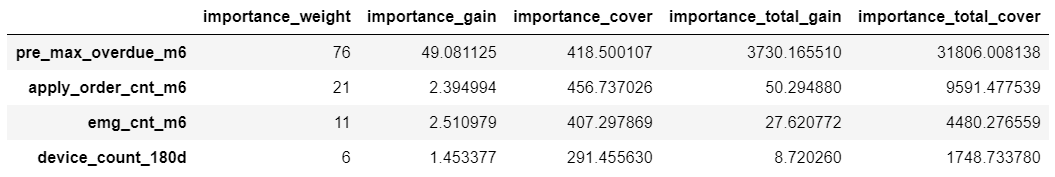• weight：在所有树中，某特征被用来分裂节点的次数；
• total_cover：total_cover表示在所有树中，某特征在每次分裂节点时处理(覆盖)的所有样例的数量；
• cover：cover = total_cover / weight，某特征平均每次分裂覆盖的样本数；
• total_gain：在所有树中，某特征在每次分裂节点时带来的总增益，即用熵或基尼不纯衡量分裂前后的信息量之差。
• gain：gain = total_gain / weight，每次分裂的增益均值；

### Shap Value

Shap是Shapley Additive explanations的缩写，即沙普利加和解释，对于每个样本模型都产生一个预测值，Shap value就是该样本中每个特征所分配到的数值。 与线性模型的加和方法类似， 假设模型基准分（通常是所有样本的目标变量的均值）为，第i个样本为，第i个样本的第j个特征为，该特征的Shap value为，则模型对样本的预测值为>0，说明该特征对目标值的预测起到了正向作用；反之，该特征使与目标预测值有相反作用。因此Shap不仅给出特征影响力的大小，也反映出每一个样本中的特征的影响力的正负性。

import shap
explainer = shap.TreeExplainer(trained_xgb) # 引用package并且获得解释器explainer
shap_values = explainer.shap_values(data[cols]) # 获取训练集data各个样本各个特征的SHAP值
y_base = explainer.expected_value # 模型的基线值ybase就是训练集的目标变量的拟合值的均值
• 单个样本的Shap值
# 挑选数据集中的第25位
j = 25
player_explainer = pd.DataFrame()
player_explainer['feature'] = cols
player_explainer['feature_value'] = data[cols].iloc[j].values
player_explainer['shap_value'] = shap_values[j]
player_explainer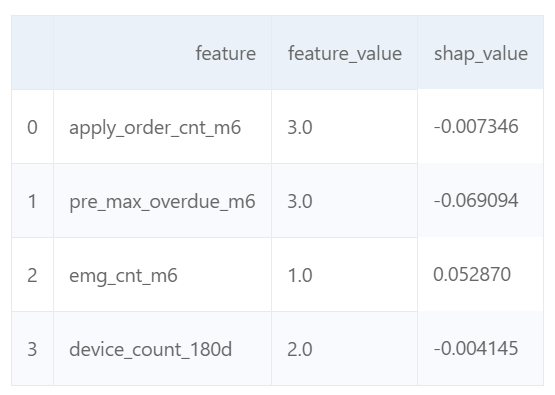shap.initjs()
shap.force_plot(explainer.expected_value, shap_values[j], data[cols].iloc[j],link='logit')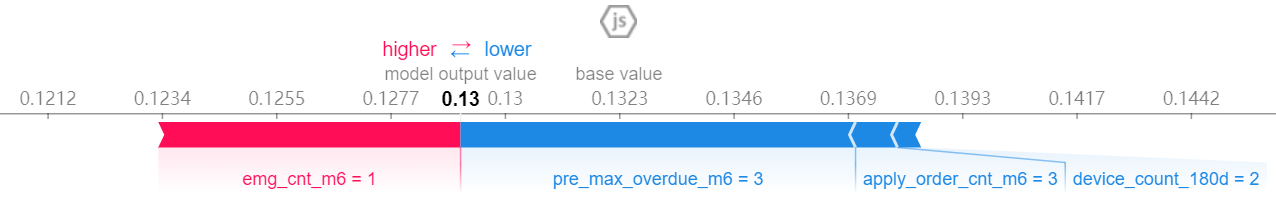• 对特征的总体分析

shap.summary_plot(shap_values, data[cols])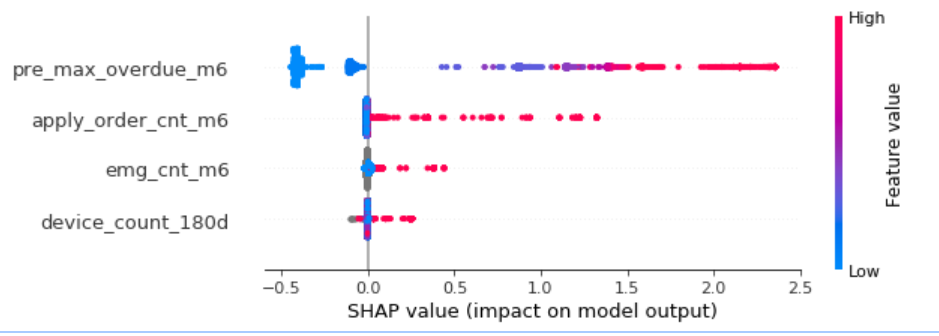shap.summary_plot(shap_values, data[cols], plot_type="bar")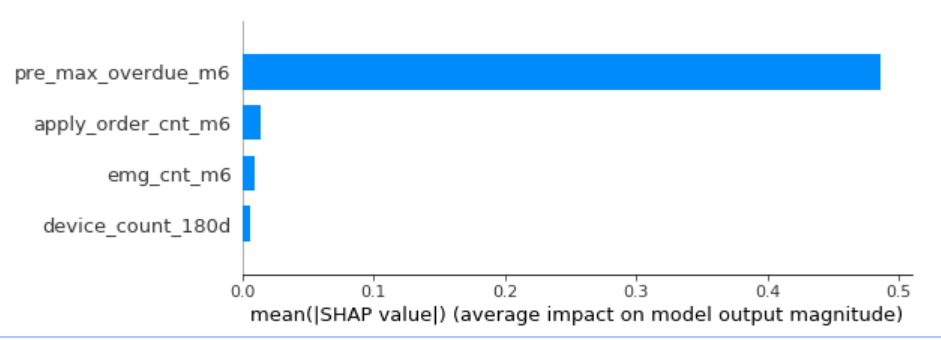• 部分依赖图Partial Dependence Plot

SHAP提供了部分依赖图的功能，纵坐标为SHAP值，横坐标为变量分布。可以看到历史逾期天数越大，对target预测为1的影响越大，当逾期天数大到一定阈值，Shap达到峰值不变，也就是基本可以判断为逾期样本。

shap.dependence_plot('pre_max_overdue_m6', shap_values, data[cols], interaction_index=None, show=False)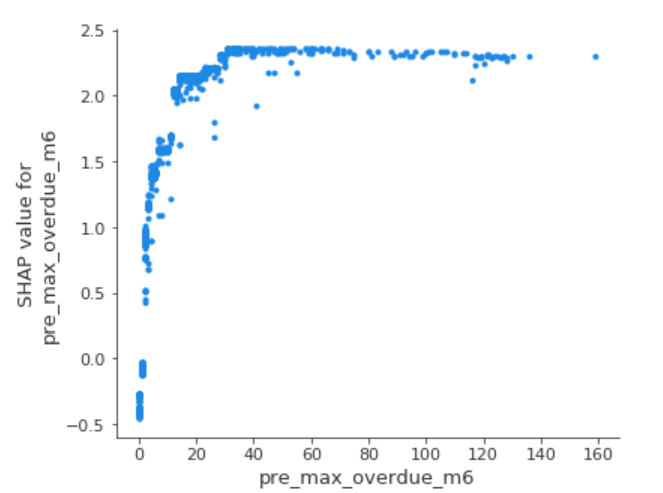07-17107102-131408
05-18943
06-027071
09-07919
10-241万+
10-254234